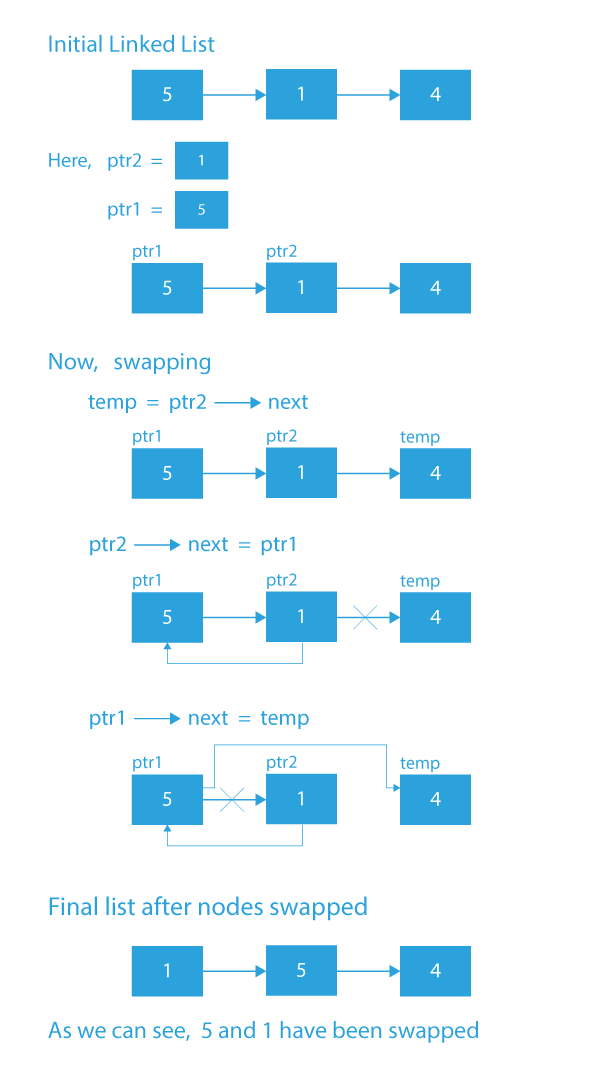# Bubble Sort For Linked List By Swapping Nodes

## Introduction

While preparing for interviews, one of the most significant concepts and data structures to grasp is the linked list. A solid understanding of Linked Lists may be a great asset in a coding interview.

So Today, we will discuss a problem based on the linked list and its implementation.

## Problem Statement

Given a singly linked list. We have to sort this list using bubble sort by swapping nodes. We need to swap the actual nodes of the linked list instead of the values.

Example

Input:

5 1 4 2 8

Output:

1 2 4 5 8

Explanation

We have the following linked list:

5 -> 1 -> 4 -> 2 -> 8

After Sorting it must be

1 -> 2 -> 4 -> 5 -> 8

## Approach :  Bubble Sort

Bubble Sort is the most basic sorting algorithm, and it operates by periodically exchanging nearby elements if they are out of order. Sorting is accomplished by going over all of the items one by one, comparing them to the next element, and exchanging them if necessary.

Here the algorithm for bubble sort remains the same, the only change that will affect the linked list is how are we performing the swap of two nodes since we need to swap actual nodes, not just the values.

### Swapping Two Nodes

This is the most tricky part here because we have to swap the nodes themselves, not the values of the nodes.

Let the two adjacent nodes swapped be p1 and p2.

• Now we'll construct two pointers, ptr1 and ptr2, and set ptr1 to point to p1 and ptr2 to point to p2.
• Next, we'll build a temporary pointer that points to the next of ptr2.
• We'll make ptr2's next point to ptr1, and ptr1's next point to temp.
• Following the above steps, the two nearby nodes p1 and p2 will be swapped.Source: prepbytes

### Code

``````/*
Time Complexity: O(N2);
Space Complexity: O(1);
*/

#include <iostream>
#include <bits/stdc++.h>
using namespace std;

class Node
{
public:
int data;
Node *next;
Node(int data)
{
this->data = data;
this->next = NULL;
}
};

{
int i = 0 ;
while(temp!=NULL)
{
i++;
temp=temp->next ;
}

return i ;
}

Node* swap(Node* ptr1, Node* ptr2)
{
Node* tmp = ptr2->next;
ptr2->next = ptr1;
ptr1->next = tmp;
return ptr2;
}

{
Node** temp;
int i, j, swapped;
Node* p1,p2;

for (i = 0; i <= length; i++)
{

swapped = 0;

for (j = 0; j < length - i - 1; j++)
{

p1 = *temp;
p2 = p1->next;

if (p1->data > p2->data)
{

/* update the link after swapping */
*temp = swap(p1, p2);
swapped = 1;
}

temp = &(*temp)->next;
}

if (swapped == 0)
break;
}
return;
}

Node *takeinput()
{
int n;
cin >> n;
Node *head = NULL, *tail = NULL;
while (n--)
{
int data;
cin>>data;
Node *newnode = new Node(data);
{
tail = newnode;
}
else
{
tail->next = newnode;
tail = newnode;
}
}
}

{
while (temp != NULL)
{
cout << temp->data << " ";
temp = temp->next;
}
cout << endl;
}

int main()
{
}``````

Input:

``5 5 1 4 2 8``

Output:

``1 2 4 5 8``

### Complexity Analysis

#### Time Complexity: O(N2)

In Bubble Sort, n-1 comparisons will be done in the 1st iteration, n-2 in 2nd iteration, n-3 in 3rd iteration and so on. So the total number of comparisons will be,

(N-1) + (N-2) + (N-3) + ..... + 3 + 2 + 1

∑ N

N(N-1)/2

i.e O(N2)

#### Space Complexity: O(1)

The algorithm only requires auxiliary variables for flags, temporary variables, and thus, the space complexity is O(1).

1. Why the time complexity of Bubble sort is O(N2)?
Time Complexity of Bubble Sort is O(N2) because for the first iteration there will be N-1 comparisons, in the second iteration, there will be N-2 comparisons and so on till we reach Nth iteration where there will be 1 Comparison. So total number of operations are ∑ N  i.e. N(N-1)/2 which is O(N2).

2. Why do we need to swap the nodes themselves?
We need to swap the nodes instead of values because in a bubble sort of any data structure we tend to swap the element of that structure. When we have an array, our element is an Integer value but here we have a linked list so our element here is a node. Moreover, swapping only values of nodes may give inaccurate results.

3. What is the time complexity of the Swap function?
Swap functions consist of 3 operations only thus it’s a constant time complexity function which is O(1).

## Key Takeaways

Bubble Sort can be applied to any data structure. The algorithm of the bubble sort remains the same but the only thing that varies is the swap function according to the kind of data structure to be sorted.